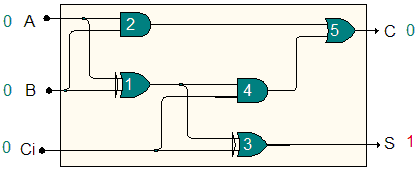Proposed by Francisco Azevedo

Model-based diagnosis can be seen as taking as input a partially parameterized structural description of a system and a set of observations about that system. Its output is a set of assumptions which, together with the structural description, logically imply the observations, or that are consistent with the observations.

Diagnosis is usually applied to combinational digital circuits, seen as black-boxes where there is a set of controllable input bits but only a set of primary outputs is visible.

The problem is to find the set S of all (minimal) internal faults that explain an incorrect output vector F (different than the modelled, predicted, output vector N), given some input vector I.

The possible faults consider the usual stuck-at fault model, where faulty circuit gates can be either stuck-at-0 or stuck-at-1, respectively outputting value 0 or 1 independently of the input.

In the example full-adder circuit below, the single faults that explain the incorrect output (instead of the expected <00>) are Gate1 stuck-at-1 or Gate3 stuck-at-1.For I = <000> and F = <10> (S=1,C=0), the diagnosis result is thus S = {{Gate1/1},{Gate3/1}} (with Gate/1 meaning Gate stuck-at-1). Each element of S is an internal malfunction (a set of faults) that is an explanation for the incorrect output.

The diagnosis problem becomes more complex when the minimal internal malfunction is not a single fault, but rather a set of faulty gates (double faults, triple faults, and so on), with complexity increasing with the cardinality of such set. We may also want to explicitly find (e.g.) double faults instead of single faults to make a problem harder.